# Gizmo Inputs

You can have up to 8 gizmo inputs into the Pynapse gizmo. These inputs can be digital signals (logic TTL) or analog signals (float or integer) that go through a Logic Conversion, such as thresholding.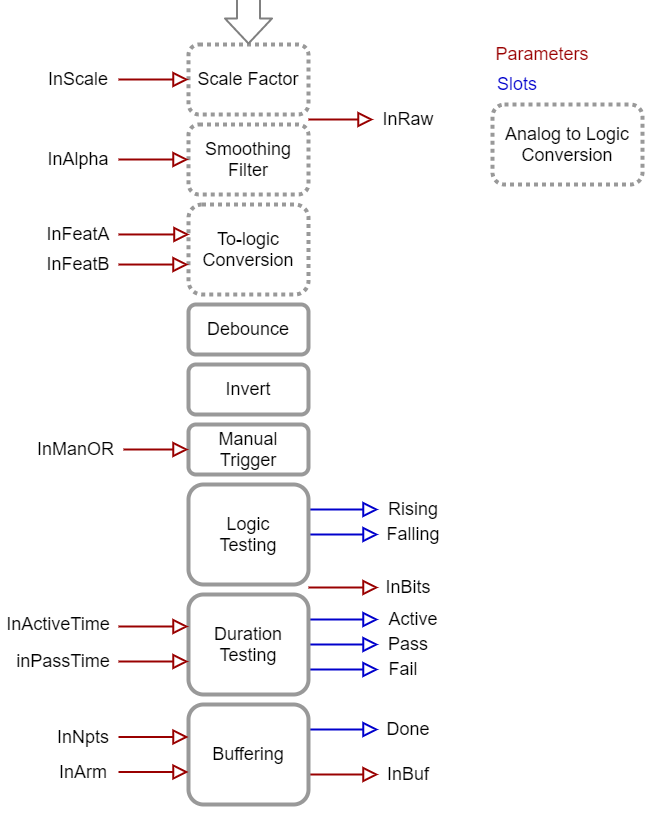Input Processor

Set the Name of the input to something that makes sense for your experiment, e.g. 'NosePoke'. This will be used throughout the Python code.

You can optionally save epoch timestamp events for each input. An integer code for the event type is stored with the timestamp. See Epoc Storage below for more information.

## Logic Conversion for Number Signals

Number input signals pass through a logic conversion so they can trigger on/off events in Pynapse.

Pre-scale is a scalar multiplied by the signal.

Smoothing is a low pass filter that removes jitter on the analog signal before logic conversion. This helps avoid a situation where the signal is quickly bouncing around the test threshold.

By default, the Test is Above, which is a simple threshold detection method to convert the number signal input into a logic signal when it goes beyond the Thresh-A value.

Epoc events are triggered on the 'rise' event of all of these tests. If the test can be true for more than 2 samples ('Strobe') then a timestamp for the 'fall' event is also stored. See Epoc Storage below for full epoc code information.

Test Type Description Duration
Above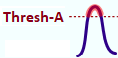Signal is above Thresh-A Strobe
Below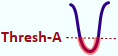Signal is below Thresh-A Strobe
Between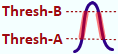Signal is between Thresh-A and Thresh-B Strobe
Outside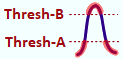Signal is outside Thresh-A and Thresh-B Strobe
Rising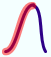Signal is increasing in value Strobe
Falling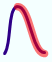Signal is decreasing in value Strobe
Peak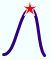Signal forms a local peak Trigger
Valley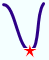Signal forms a local valley Trigger
Tip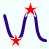Signal forms a local peak or valley Trigger
Rise Thru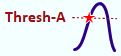Signal rises through Thresh-A from below Trigger
Fall Thru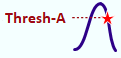Signal falls through Thresh-A from above Trigger
Pass Thru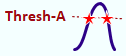Signal passes through Thresh-A from above or below Trigger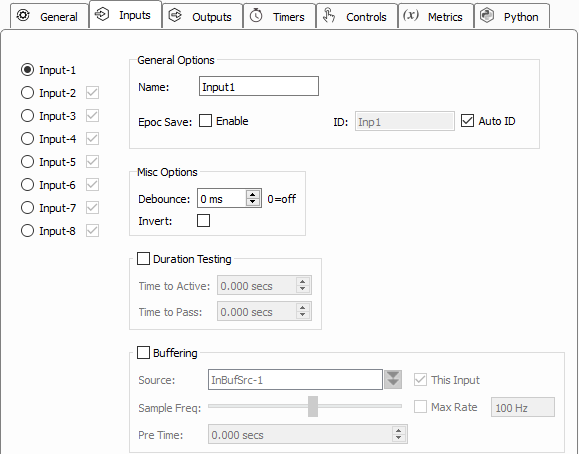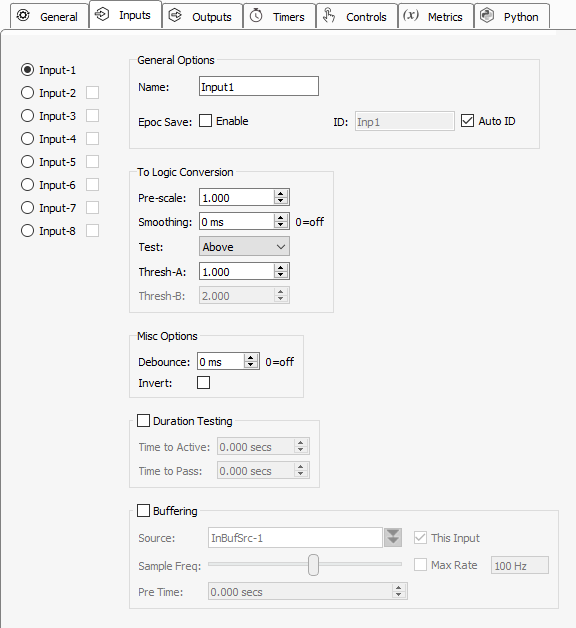Invert and Debounce are typically used if the input is coming directly from a digital input on the RZ processor. Debounce is the amount of time the input has to settle before its new state is used. This is useful for lever presses or hardware button presses which can 'bounce' on contact and trigger several rapid artificial events before making solid contact.

## Slot Methods for Responding to Input States

These input slots capture status information about the inputs. They are available as method definitions inside Pynapse states for each input. Write a method with this name to react to the corresponding event.

Slot name Operation Event
s_Input1_rise() Status input changed to true
s_Input1_fall() Status input changed to false
s_Input1_active() Duration input passed the 'Time to Active' duration test (see Duration Testing)
s_Input1_pass() Duration input passed the 'Time to Pass' duration test (see Duration Testing)
s_Input1_fail() Duration input failed the 'Time to Pass' duration test, after passing 'Time to Active' (see Duration Testing)
s_Input1_done() Buffer input buffer is full and ready to be read (see Buffering)

Note

'Input1' is default name of the first input. The name of each slot method gets replaced with the name of your actual input, so if you name the input 'NosePoke' then s_NosePoke_rise() is an available slot

Example

Move through behavioral states based on status of MyInput

``````class PreTrial:
def s_MyInput_rise():
p_State.switch(StartTrial)

class StartTrial:    # StateID = ?
def s_MyInput_active():
p_State.switch(ActiveState)

def s_MyInput_fall():
p_State.switch(PreTrial)

class ActiveState:    # StateID = ?
def s_MyInput_pass():
p_State.switch(PassState)

def s_MyInput_fail():
p_State.switch(FailState)
``````

## Duration Testing

The inputs can use built-in duration testing. In this example, the button has to be pressed for 600 ms to get to the 'Active' state, and another 400 ms for it to 'Pass'. This timing happens on the hardware and the `active`, `pass` and `fail` slots are triggered in Pynapse.

Time to Active not reached by Input.
No trial is initiated.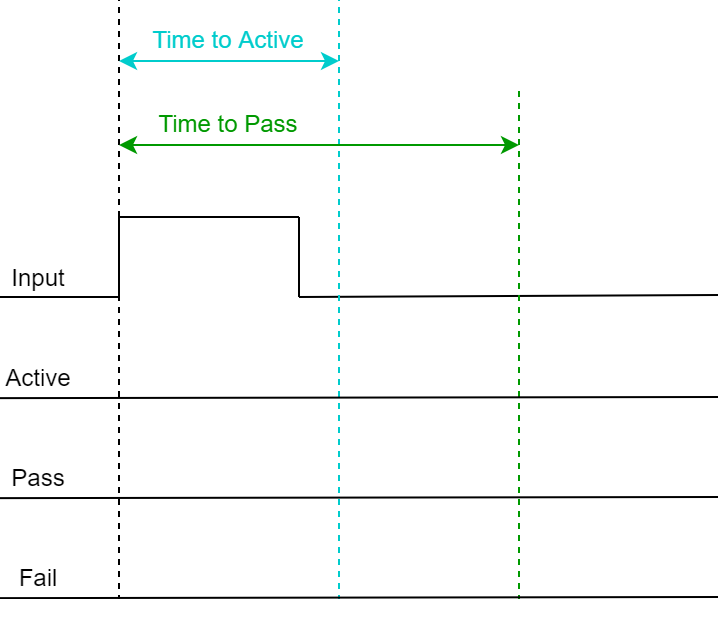Time to Active reached by Input, so 'Active' trigger fires.
Time to Pass was not reached by Input, so the 'Fail' trigger fires when Input is released.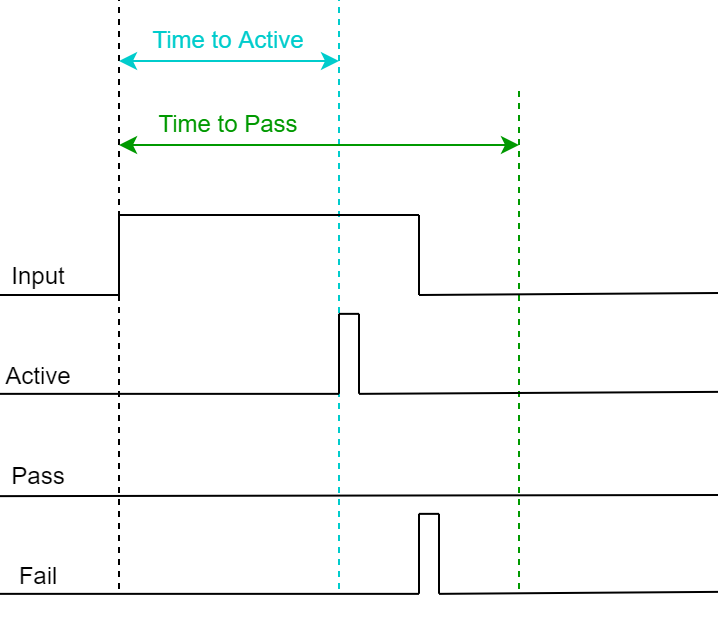Time to Active reached by Input, so 'Active' trigger fires.
Time to Pass also reached by Input, so 'Pass' trigger fires.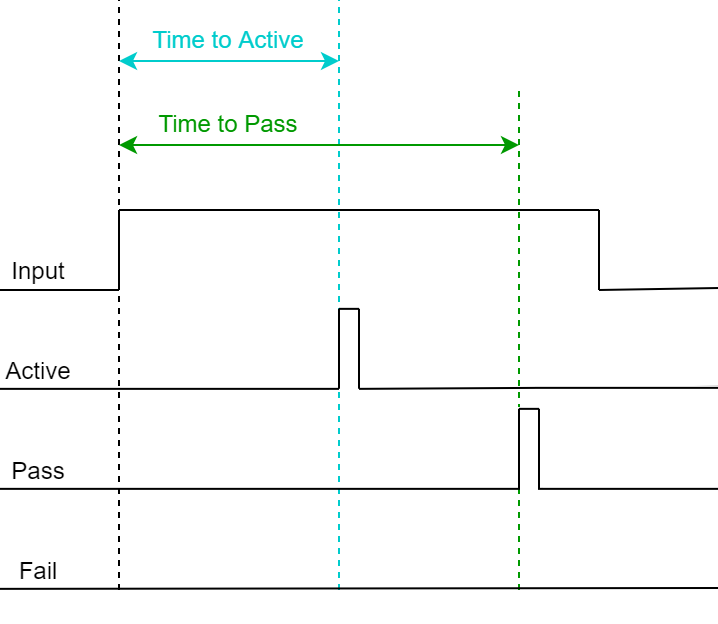## Epoc Storage

Epoc events are triggered on the 'rise' event of the input and a timestamp and value of 3 is stored in the data tank. If the input is true for more than 2 samples then the 'fall' event is also timestamped and stored, with a value of 4.

The full state of the input, including duration test results, is captured in the integer code:

b6 b5 b4 b3 b2 b1 b0
Done Fail Pass Active Fall Rise True

Example values of the epoc event:

Event Value Binary Representation
Rise 3 0x0000011
Fall 4 0x0000100
Active 9 0x0001001
Pass 17 0x0010001
Fail 36 0x0100100
Done 64 0x1000000

## Buffering

Buffering lets you save a small snippet of data in hardware memory and read it into Pynapse. When the input switches to `true` ('rise' trigger) the buffer is captured. When buffering is finished it fires the `done` trigger.

You can connect the buffer signal source can be any single channel signal in your experiment, including the input signal. Even though the inputs are all converted to digital signals for logic tests, you can still trigger Pynapse to buffer up the original analog signal and then read that into Python for online analysis. For example, you can do a threshold detection on a signal and save the snippet around the threshold crossing, and do something with this in Pynapse.

Example

Display a 1000 sample buffer when triggered.

``````%matplotlib

import matplotlib.pyplot as plt

class Always:   #StateID = 0

def s_Mode_standby():
# set up the buffer and plot
p_Input.MyInput2.setBufferSize(1000)
p_Input.MyInput2.armBuffer()
plt.plot()

def s_MyInput_done():
# get buffer
arr = p_Input.MyInput2.getBuffer()

# plot buffer contents
plt.plot(arr)
``````

## Methods

All input methods have the form `p_Input.{INPUT_NAME}.{METHOD}`. Type `p_` in the Pynapse Code Editor and let the code completion do the work for you.

### Buffer operations

Read a triggered snippet of memory from the hardware.

#### setBufferSize

``````p_Input.MyInput.setBufferSize(npts)
``````

Change the number of samples to store in the buffer.

Inputs Type Description
npts integer Number of samples to buffer

Important

This call must always be made if using a buffer.

Example

Initialize the buffer size before the recording starts.

``````class Always:   #StateID = 0

def s_Mode_standby():
# set up the buffer
p_Input.MyInput.setBufferSize(1000)
p_Input.MyInput.armBuffer()
``````

#### armBuffer

``````p_Input.MyInput.armBuffer()
``````

Let the buffer accept a trigger and fill with new data.

Important

This call must always be made if using a buffer.

Example

Arm the buffer when the experiment first runs.

``````class Always:   #StateID = 0

def s_Mode_standby():
# set up the buffer
p_Input.MyInput.setBufferSize(1000)
p_Input.MyInput.armBuffer()
``````

#### disarmBuffer

``````p_Input.MyInput.disarmBuffer()
``````

Stop the buffer from loading again. Use this to avoid overwriting buffer data before you've had a chance to read it with `getBuffer`.

Example

Prevent the hardware buffer from triggering/ loading new data while you read it.

``````p_Input.MyInput.disarmBuffer()
arr = p_Input.MyInput.getBuffer()
p_Input.MyInput.armBuffer()
``````

#### getBuffer

``````arr = p_Input.MyInput.getBuffer(npts=0, offset=0)
``````
Inputs Type Description
npts integer Number of samples to read (0=all)
offset integer Starting index in buffer to read from (0-based)
Returns
array number Buffer contents as python list
Example

Capture the MyInput buffer when the 'done' trigger fires.

``````class Always:   #StateID = 0

def s_Mode_standby():
# set up the buffer
p_Input.MyInput.setBufferSize(1000)
p_Input.MyInput.armBuffer()

def s_MyInput_done():
# get buffer
arr = p_Input.MyInput.getBuffer()
print(arr)
``````

### Duration Settings

#### setActTime

``````p_Input.MyInput.setActTime(acttime_sec)
``````

Override the duration test 'Time to Active' setting.

Inputs Type Description
acttime_sec float Time to Active, in seconds
Example

Modify the timing test based on performance.

``````def s_State_enter():
# if more than 5 successful trials, increase the time to active by 50 ms.
if p_Metric.success.read() > 5:
p_Metric.active_time.inc(delta=0.05)
``````

#### setPassTime

``````p_Input.MyInput.setPassTime(passtime_sec)
``````

Override the duration test 'Time to Pass' setting.

Inputs Type Description
passtime_sec float Time to Pass, in seconds
Example

Modify the timing test based on performance.

``````def s_State_enter():
# if more than 5 successful trials, increase the time to pass by 50 ms.
if p_Metric.success.read() > 5:
p_Metric.pass_time.inc(delta=0.05)
``````

### Manual Control

Manual turn inputs on, off, or pulse during runtime. Useful for debugging.

#### manualOn

``````p_Input.MyInput.manualPulse()
``````

Manually turn on the input.

Example

Turn on the input when entering a state.

``````def s_State_enter():
p_Input.MyInput.manualOn()
``````

#### manualOff

``````p_Input.MyInput.manualPulse()
``````

Manually turn off the input.

Example

Turn off the input when exiting a state.

``````def s_State_exit():
p_Input.MyInput.manualOff()
``````

#### manualPulse

``````p_Input.MyInput.manualPulse()
``````

Manually pulse the input.

Example

Pulse the input when entering a state.

``````def s_State_enter():
p_Input.MyInput.manualPulse()
``````

### Number Conversion Settings

Override the feature settings applied to the input signal for logic conversion at runtime.

#### setFeatureThresholds

``````p_Input.MyInput.setFeatureThresholds(thresh_A, thresh_B)
``````

Modify the threshold settings for the logic conversion.

Inputs Type Description
thresh_A float Threshold (in V) for the Thresh-A parameter
thresh_B float Threshold (in V) for the Thresh-B parameter
Example

Modify the lever force requirement based on performance.

``````def s_State_enter():
# if more than 5 successful trials, increase the force required by 0.05
if p_Metric.success.read() > 5:
p_Metric.thresh_A.inc(delta=0.05)
``````

#### setScale

``````p_Input.MyInput.setScale(scalefactor)
``````

Change the scale factor applied to the signal before it goes through the logic conversion.

Inputs Type Description
scalefactor float Scale factor applied to signal before logic conversion
Example

Modify the scale factor based on a run-time Control.

``````class Always:   #StateID = 0

# set input scale factor to value of the SmoothCtrl slider at runtime
def s_SmoothCtrl_change(value):
p_Input.MyInput.setScale(value)
``````

#### setSmoothing

``````p_Input.MyInput.setSmoothing(tau_sec)
``````

Change the smoothing filter applied to the input signal before it goes through the logic conversion.

Inputs Type Description
tau_sec float Time constant of the low-pass smoothing filter, in seconds (0=off)
Example

Modify the smoothing filter based on a run-time Control.

``````class Always:   #StateID = 0

# set smoothing 'tau' to value of the TauCtrl slider at runtime
def s_TauCtrl_change(value):
p_Input.MyInput.setSmoothing(value)
``````

### Status

Get information on the current state of the input.

#### isOn

``````p_Input.MyInput.isOn()
``````

Returns true if the input is currently true.

Example

When entering a state, check if an input is already true.

``````def s_state_enter():
if p_Input.MyInput.isOn():
print('MyInput is on')
else:
print('MyInput is off')
``````

#### isOff

``````p_Input.MyInput.isOff()
``````

Returns true if the input is currently false.

Example

When entering a state, check the status of the input.

``````def s_state_enter():
if p_Input.MyInput.isOff():
print('MyInput is off')
else:
print('MyInput is on')
``````

#### getRawInput

``````p_Input.MyInput.getRawInput()
``````

Read the current value of an input. If it is a number, the raw input into the Pynapse gizmo after scale factor is applied but before any feature detection.

Example

When a threshold is crossed, check the current value of the signal.

``````def s_MyInput_rise():
print(p_Input.MyInput.getRawInput())
``````

#### getStatusBits

``````p_Input.MyInput.getStatusBits()
``````

Read the current state of an input as a bitwise integer value. Bit order is:

`Done | Fail | Pass | Active | Fall | Rise | True`

Used by the Pynapse polling loop.